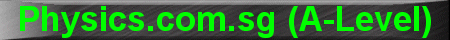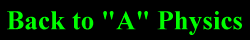(New tips are continually added to these pages.  Check back in a few months' time for more)

TOPIC 7:     Oscillations and Resonance

Tip 1:

Although not officially in the syllabus, the following equations should be memorised.  The alternative, ie. to derive on the spot, may be difficult for the average student.

For a spring-mass system, the natural period of oscillation is given by:

T = 2p Ö(m/k) = 2p Ö(e/g).

where m is the mass of the object attached to the spring; k the spring constant; e the extension of the spring; and g the acceleration due to gravity.

Why is m/k = e/g?  That's because mg = ke.

For a pendulum system, the natural period of oscillation is given by:

T = 2p Ö(l/g).

where l is the length of the string and g the acceleration of free fall.

Example 1:

For a spring-mass system, if the the spring is replaced by 2 springs of the same kind in series, and the mass is doubled, what happens to the period of oscillation?

Answer: For 2 springs in series, the spring constant is halved.  Since m is doubled and k is halved, m/k will quadruple; and Ö(m/k) will double.  Therefore, the period T will double.

Example 2:

If I relocate both a pendulum and a spring-mass system to the moon, where the acceleration due to gravity is 1/6 that on Earth, what happens to the period in each case?

Answer:  the period of the pendulum will be Ö6 times that on Earth, while that of the spring-mass system will remain unchanged.

Reason: since g becomes 1/6 times its original value, the ratio l/g becomes 6 times its original value.  Therefore, Ö(l/g) becomes Ö6 times its original value.

But what about the spring-mass system? Well, in this case, the mass m is constant (mass does not change); the spring constant k is constant.  Hence period T = 2p Ö(m/k) is also constant.

Trick Question:

But for a spring-mass system, isn't period T also = 2p Ö(e/g) ?  So shouldn't the period also be affected by g as well?

Answer: on the moon, g is 1/6 times, but the extension of the spring also becomes 1/6 times since the mass now weighs only 1/6 of its weight on Earth.  So the 1/6 in the numerator cancels out with the 1/6 in the denominator, and the period remains unchanged.